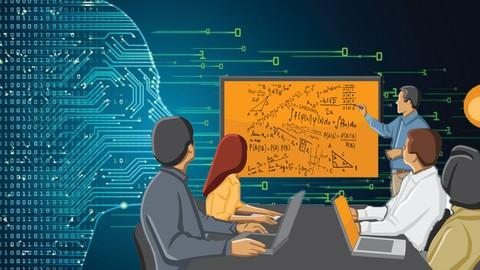# The Complete Mathematics Software Developer Course [100% OFF UDEMY COUPON]This course covers all Mathematics needed to become Software Developer. Here we will discuss Linear Algebra, Modern Analysis, Mathematical Logic, Number Theory and Discrete Mathematics. By the end of this course you will be able to analyze and describe computer science concepts and methods. This course is a great opportunity for you to gain deep understanding of all processes a executed in the computer system when programming. The specific objectives of the course are the following:

Learn how to apply proof techniques to your computer program. Learn encrypting and decrypting messages with Number Theory. Learn how the software development is related to Discrete Mathematics and Digital Electronics. Understand how to use mathematical tools to properly analyze any computer algorithm.Learn how to apply Calculus, Probability Theory and Linear Algebra while computing. Understand how to apply Lambda Calculus to Functional Programming.

Discrete mathematics is the study of mathematical structures that are fundamentally discrete rather than continuous. In contrast to real numbers that have the property of varying “smoothly”, the objects studied in discrete mathematics – such as integers, graphs, and statements in logic – do not vary smoothly in this way, but have distinct, separated values.Discrete mathematics therefore excludes topics in “continuous mathematics” such as calculus or Euclidean geometry. Discrete objects can often be enumerated by integers. More formally, discrete mathematics has been characterized as the branch of mathematics dealing with countable sets (finite sets or sets with the same cardinality as the natural numbers). However, there is no exact definition of the term “discrete mathematics.” Indeed, discrete mathematics is described less by what is included than by what is excluded: continuously varying quantities and related notions.

## What you'll learn :

1. Proof Techniques. Mathematical Induction and Recursion Theory.
2. Mathematical Logic. Propositional and First Order Calculus. Model Theorem.
3. Programs verifications and Model Checking
4. Linear Algebra. Matrix Theory in Computer Science.
5. Boolean Algebra and its applications in Digital Electronics.
6. Lambda Calculus as a Foundation of Functional Programming
7. Number Theory and Encryption.
8. Modern Statistics and Probabilistic Methods in Computer Science.
9. Functional Analysis and the efficiency of computer algorithms Decision Theory

## Apply this Coupon :

FREE_SUMMER

If Coupon is Expired/Invalid !!!

Possible Reasons!

• One coupon can last maximum for 2 days. Your are Late
• Author revoked the coupon. Author have rights to revoke the coupon any time.You missed it Bad Luck!!!

But Don’t worry , if you missed it, join our groups to receive all coupon instantly whenever it is posted.